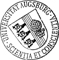Central Limit Theorem for the Integrated Squared Error of the Empirical Second-Order Product Density and Goodness-of-fit Tests for Stationary Point Processes

• Spatial point processes are mathematical models for irregular or random point patterns in the d-dimensional space, where usually d=2 or d=3 in applications. The second-order product density and its isotropic analogue, the pair correlation function, are important tools for analyzing stationary point processes. In the present work we derive central limit theorems for the integrated squared error (ISE) of the empirical second-order product density and for the ISE of the empirical pair correlation function for expanding observation windows. The proof techniques are based on higher-order cumulant measures and the Brillinger-mixing property of the underlying point processes. The obtained Gaussian limits are used for constructing asymptotic goodness-of-fit tests for checking point process hypotheses even in the non-Poissonian case.

• Dokument_1.pdfAuthor: Stella Veronica David, Lothar HeinrichGND urn:nbn:de:bvb:384-opus4-11890 https://opus.bibliothek.uni-augsburg.de/opus4/1466 Preprints des Instituts für Mathematik der Universität Augsburg (2010-17) Preprint English Universität Augsburg 2010/12/06 second-order analysis of point processes; pair correlation function; Brillinger-mixing; cumulant measures; model specification test Räumliche Statistik; Zufälliger Punktprozess; Stationärer Punktprozess; Asymptotik; Anpassungstest; Zentraler Grenzwertsatz Mathematisch-Naturwissenschaftlich-Technische Fakultät Mathematisch-Naturwissenschaftlich-Technische Fakultät / Institut für Mathematik Mathematisch-Naturwissenschaftlich-Technische Fakultät / Institut für Mathematik / Lehrstuhl für Stochastik und ihre Anwendungen 5 Naturwissenschaften und Mathematik / 51 Mathematik / 510 MathematikDeutsches Urheberrecht mit Print on Demand